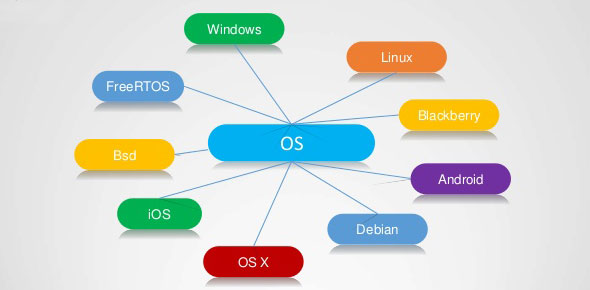# Test Your Knowledge On This JCL Quiz Questions

45 Questions | Attempts: 3818
ShareSettings• 1.
If  we need to code  a more than 255 STEPS in a job
• A.

A. We need to split jcl into two jcl, at the end of the first jcl check the cc and initiate the second jcl.

• B.

B.We can code one more steps in the same jcl itself

• C.

C.We can’t able to code a new step

• D.

D.None of the above

• 2.
Maximum datasets exist within one GDG
• A.

A.120

• B.

B.144

• C.

C.225

• D.

D.255

• 3.
EMPTY parameter in GDG creation defines
• A.

A.If GDG reaches a Maximum limit, then cataloged all the existing generation

• B.

B.If GDG reaches a Maximum limit, then cataloged all the oldest generation

• C.

C.If GDG reaches a Maximum limit, then uncataloged all the oldest generation

• D.

D.If GDG reaches a Maximum limit, then uncataloged all the existing generation

• 4.
In IDCAMS – ‘AMS ‘ stands for
• A.

A.Access Method System

• B.

B.Access Method Services

• C.

C.Access Method Subsystem

• D.

D.Access Method Submission

• 5.
Suppose the current version of dataset is ‘mydata.lib.test.Gooo1.V00’ and the next version would be
• A.

A.mydata.lib.test.Gooo1.V01

• B.

B.mydata.lib.test.Gooo1.V02

• C.

C.mydata.lib.test.Gooo1.V10

• D.

D.mydata.lib.test.Gooo2.V00

• 6.
GDG means
• A.

A.Generation data groups

• B.

B.Grouping generation data

• C.

C.Grouping data generation

• D.

D.Grouping and data groups

• 7.
………………….  used to pass a value to procedure parameters.
• A.

A.Disp parameter

• B.

B.Positional parameter

• C.

C.Symbolic parameter

• D.

D.Space parrameter

• 8.
Once the procedure is written, we can’t able to change procedure statements according to our reuirements
• A.

True

• B.

False

• 9.
System library which has a place for catalog procedure called
• A.

A.SYS1.PROCLIB

• B.

B.SYS1.PROC.LIB

• C.

C.SYS1.CATGPROC.LIB

• D.

D.SYS1.PROC.CATGLIB

• 10.
Instream procedure should be defined before any EXEC statements
• A.

True

• B.

False

• 11.
Maximum number of Instream procedure you can in any job is
• A.

A)15

• B.

B)59

• C.

C)120

• D.

D)144

• 12.
……………………….. is used to dump the contents of various registers variables, dataset accessed and the nucleus at the time of abnormal terminal.
• A.

A.SYSIN

• B.

B.SYSOUT

• C.

C.SYSDUMP

• D.

D.SYSABEND

• 13.
Syntax1 //myjob job notify=&sysuid              //s1 exec pgm=cobprog             //sysin dd *             /* syntax1 //myjob job notify=&sysuid              //s1 exec pgm=cobprog             //sysin dd  data             /* Which syntax is used pass data to program
• A.

A)Syntax1

• B.

B)Syntax2

• C.

C)Both

• D.

D)Only syntax1 not syntax2

• 14.
Maximum Limit for concatenating dataset for PS and PDS
• A.

A)16 and 255

• B.

B)256 and 20

• C.

C)255 and 16

• D.

D)20 and 255

• 15.
If we need to test the program without using actual datasets, then we can use
• A.

A)DUMMY

• B.

B)DSN = NULLFILE

• C.

C)Both

• D.

D)None

• 16.
PASS parameter of DISP is used in
• A.

A)Status

• B.

B)Normal disposition

• C.

C)Abnormal disposition

• D.

D)None of these

• 17.
Normal Disposition is happened upon successful execution of job step
• A.

True

• B.

False

• 18.
To allow a job or step to use the maximum amount of time,code Time = Maximum
• A.

True

• B.

False

• 19.
//second job Time=3 //step1 exec pgm=c,time=2 //step2 exec pgm=d,time=2 Which of the following is correct about  the above statement
• A.

A)Both the step should execute within 3 minutes

• B.

B)Both the step should execute within 2 minutes

• C.

C)Both the step should execute within 5 minutes

• D.

D)Both the step should execute within 7 minutes

• 20.
How will u give a job or step an unlimited amount of time
• A.

A) TIME = 1040

• B.

B) TIME = 1440

• C.

C) TIME = NOLIMIT

• D.

D) TIME = UNLIMIT

• 21.
//Myjob job notify=&sysuid,time=(20,30) //step1 exec pgm=cob1 //step2 exec pgm=cob1 //step3 exec pgm=cob1 Which of the following is correct about  the above statement
• A.

A.First step should execute within 20min.,second step should execute witnin 10 min. and no time limit for step3

• B.

B)Time Parameter specified in jobcard only,so it will not affect the steps.

• C.

C)Each step in this job should complete its task within 20min. 30sec.

• D.

D)All step in this job should complete its task within 20min. 30sec.

• 22.
//Myjob  job  notify=&sysuid //                   cond=(4,gt) //step1 exec pgm=cobp1 //step2 exec pgm=cobp2 What will happen in the above program
• A.

A. RC in step1 is >4 ,step2 will executes

• B.

B. RC in step1 is >4 ,step2 will not bypassed

• C.

C. RC in step1 is >4 ,step2 will bypassed

• D.

D. step1 and step2 executes regardless of condition

• 23.
Condition Code is in the range of
• A.

A)between 0 and 4096 except 2000

• B.

B)between 0 and 4096

• C.

C)between 0 and 4095 except 2000

• D.

D)between 0 and 4095

• 24.
All the available storage is assigned to the job or jobstep,if REGION is coded as 0K or 0M
• A.

True

• B.

False

• 25.
Parameter can code in both Job and EXEC statements
• A.

COND

• B.

REGION

• C.

TIME

• D.

All the above

• E.

Only a and c

## Related TopicsBack to top
×

Wait!
Here's an interesting quiz for you.